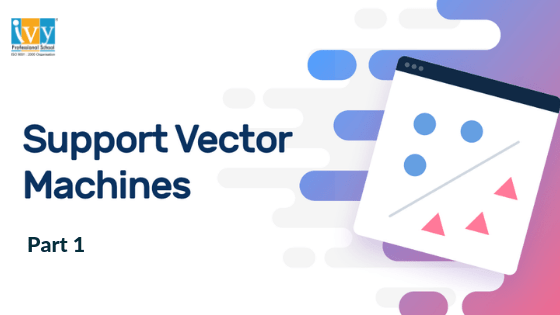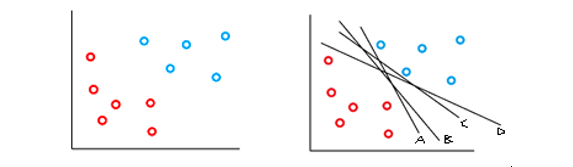# Beginners Guide to Support Vector MachinesSupport Vector Machine (SVM) is a supervised Machine Learning algorithm that performs classification tasks by constructing hyperplanes or classifiers in a multidimensional space that separates the data into two different classes. SVM supports both regression and classification tasks and can handle multiple continuous and categorical variables.

In the diagram below you can see that there are two different classes of data( Blue and Red ) .You can separate them with several separating lines such as A, B, C and  D. But there must be a single separating line or hyperplane that classifies the two classes best. So, the question is: How to choose the ultimate classifier?First it is necessary to know a few terms:

Data that can be separated by a line or a simple hyperplane is known as linearly separable data. Now those hyperplanes that can linearly separate the data are known as linear classifiers.

If the training data is linearly separable, we can obtain two parallel hyperplanes that separate the two classes of data, so that the distance between them is maximized. The region bounded by these two hyperplanes is called the “margin“, and the maximum margin hyperplane is the hyperplane that lies halfway between them. In the diagram below the line labeled as separation line is the maximum margin hyperplane.

The data points that lie closest to the hyperplanes are called Support vectors. They are the data points most difficult to classify because they lie at the boundaries of the margin and acts a support to maximize the margins.In this example we have two separation lines for the data. But, is there a good reason to choose one over another?

This question can only be answered by the margins. From the diagram it is very clear that the margin on the diagram of the right side is larger. So the hyperplane on the right side maximizes the distance between nearest data points. The aim of SVM is to choose the hyperplane in such a way that maximizes the margin between the two different classes of the dataset.

We need to remember that a classifier is not worth when the two classes seem to run too close to each other and when we run this same classifier through test data, there are high chances of misclassification. So the objective is to maximize the margin, minimize the risk involved in it and thus generalize it well on test data.

So, from the above example we can conclude that ‘B’ should be the ultimate separating line between the two classes. Now, let us take another situation. Look at the data points below; can you classify the data with a linear classifier without any misclassification?No, it is not that easy. So instead of using a linear classifier, why don’t we use a nonlinear separating line as below?The nonlinear classifier works very well to classify them and more importantly without any misclassifications.

Now, earlier I mentioned in the introduction that svm algorithm works on classification problems in multidimensional space. These multiple or ‘n’ dimensions are nothing but the number of features in the data set. And the hyperplane just looks like a thin sheet of paper that separates the two classes in a similar way as the diagram below.Ivy Pro School is India’s Top Ranked premier Data Science Institute with courses in Machine Learning, Python, R, SAS, Hadoop, Tableau and other latest Technologies in Data Science. Call us at 9748441111 for more information.